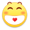# C++之运算符重载

C++引入引用的概念后， 表达式可以被赋值的现象就出现了， 有的表达式可以被赋值，有的表达式则不可以。

## 1、重载规则

+-*/%^& ~
!,=<>>=<=++--
<<>>==!=&& +=-=
%=^=&= *=<<=>>=[]()
->->*newnew[]deletedelete[]

.成员访问运算符
.*成员指针访问运算符
::域运算符
sizeof长度运算符
?:条件运算符

=赋值运算符
[]下标运算符
()函数运算符
->间接成员访问
->*间接取值访问

## 2、operator++()

``````#include <iostream>
using namespace std;
class Complex
{
public:
Complex(float x=0, float y=0)
:_x(x),_y(y){}
void dis()
{
cout<<"("<<_x<<","<<_y<<")"<<endl;
}

friend Complex & operator++(Complex& c);

private:
float _x;
float _y;
};

Complex & operator++(Complex& c)
{
c._x++;
c._y++;
return c;
}``````

``````using namespace std;
class Complex
{
public:
Complex(float x=0, float y=0)
:_x(x),_y(y){}
void dis()
{
cout<<"("<<_x<<","<<_y<<")"<<endl;
}
friend const Complex operator++(Complex &c,int);
private:
float _x;
float _y;
};
const Complex operator++(Complex &c,int)
{
Complex t(c._x,c._y);
c._x++;
c._y++;
return t;
}``````

## 3、自定义类型-转化构造函数

``````class 目标类
{
目标类(const 源类型 & 源类对象引用)
{
根据需求完成从源类型到目标类型的转换
}

};``````

``````#include <iostream>
#include <stdlib.h>
#include <time.h>
using namespace std;
//转化构造函数的本质， 也是构造函数
class Point2D
{
friend class Point3D;
public:
Point2D(int x=0, int y=0 )
:_x(x),_y(y){}
private:
int _x;
int _y;
};

class Point3D
{
public:
Point3D(int x=0, int y=0 ,int z=0)
:_x(x),_y(y),_z(z){}
Point3D(const Point2D & d2)//自定义类型-转化构造函数
{
this->_x = d2._x;
this->_y = d2._y;
this->_z = rand()%100;
}
void dumpFormat()
{
cout<<"("<<_x<<","<<_y<<","<<_z<<")"<<endl;
}
private:
int _x;
int _y;
int _z;
};

void foo(Point3D d3)
{
d3.dumpFormat();
}

int main()
{
srand(time(NULL));
Point2D d2(10,100);
Point3D d3 = d2;
d3.dumpFormat();
foo(d2);
return 0;
}``````

explicit 关键字

``````//上面代码中的这行前边加上这个关键字之后
explicit Point3D(const Point2D & d2);
//在main函数中
int main()
{
Point2D d2(10,100);
Point3D d3 = d2;// 加 explicit 后 编不过
return 0;
}
``````

### 自定义类型-操作符函数转化

``````class 源类
{
operator 目标类(void)
{
return 目标类构造器(源类实参);
}
}``````

## 4、仿函数 Functor

``````class Pow
{
public:
int operator()(int i)
{
return i*i;
}
double operator ()(double d)
{
return d*d;
}
};
int main()
{
Pow pow;
int i = pow(4);//pow.opreator()(4); pow()4;
return 0;
}``````

## 5、再论默认

• 构造函数
• 拷贝构造函数
• 拷贝赋值函数
• 析构函数

``````operator,
operator&
operator&&
operator*
operator->
operator->*
operator new
operator delete``````

Last modification：November 1st, 2019 at 02:18 pm1.不错，学习了。